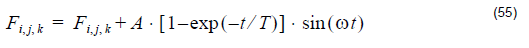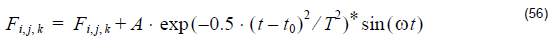# Point Source

Compatibility:

This version of OptiFDTD includes a new type of excitation – Point Source. Point
source can also be referred to as a radiation source. For this new source, the input wave is only functional with one field component at one single point. You can specify which field component is required to add this source.

In the case of CW, the point source is expressed as:where (i,j,k) is the user-specified position and F is the user specified component, A
is the point source amplitude, T is the time delay and w is the angle frequency. Fi, j, k
represents the FDTD simulation results, and the last term in the equation is the
enforced point source excitation.

For the GMCW, point source is expressed aswhere t0 is time offset and T is the half width.

Note:

• Point sources can work with other kinds of source (such as input plane source).
• Point sources can be either continuous wave (CW) or Gaussian modulated continuous wave (GMCW).
• Point sources works for both 2D and 3D simulations.
• Multiple point sources can be utilized in OptiFDTD.
• When Point source is used as the key input. The normalized power calculation will be disabled in analyzer